# HDL Implementation of Digital Predistorter with LMS Coefficient Estimation

This example shows how to implement a digital predistorter (DPD) with least mean squares (LMS) based coefficient estimation, which is optimized for HDL code generation and hardware implementation. This example expands on the HDL Implementation of Digital Predistorter example for computing DPD coefficients on an FPGA instead of a processor.

This example contains two Simulink® models.

• `HDLDPDwithLMSCoeffExample` — Use this model to perform predistortion using an adaptive LMS coefficient estimator that estimates coefficients dynamically. This model replaces the C/C++ code generatable `RPEM Coeff Estimation` subsystem of the HDL Implementation of Digital Predistorter example with an HDL-compatible `LMS Coefficient Estimator` subsystem.

• `HDLDPDwithPreCalculatedCoeffExample` — Use this model to perform predistortion using pre-calculated coefficients.

This example supports the hardware-friendly interface for the Xilinx® Zynq® UltraScale™ RFSoC ZCU111 evaluation board, which uses RF data converter. These example models support `Normal` and `Accelerator` simulation modes. For more information about DPD, see Adaptive DPD Design.

Run this command to open the high-level architecture of the HDL DPD with LMS coefficient estimation.

```modelname = 'HDLDPDwithLMSCoeffExample'; open_system(modelname); ```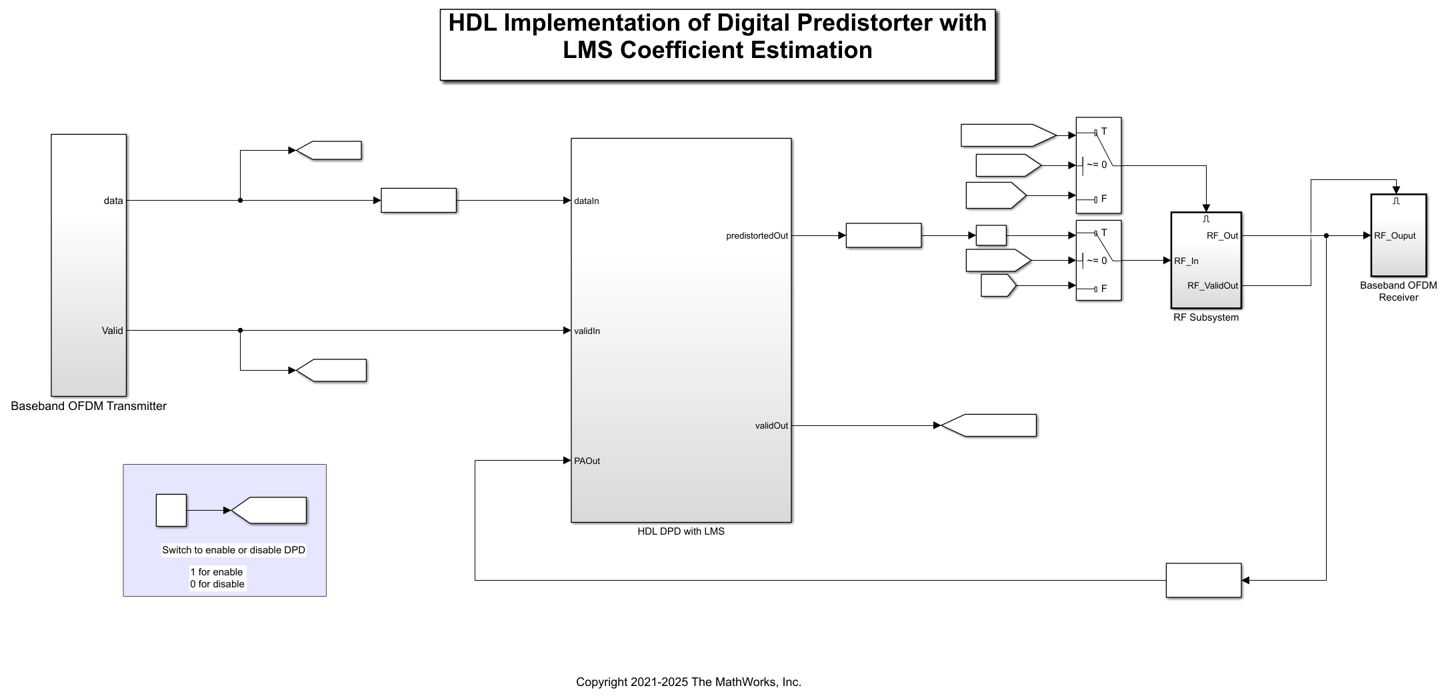### Model Architecture

The `Baseband OFDM Transmitter` subsystem generates a 16 bit complex baseband orthogonal frequency division multiplexed (OFDM) signal at a sample rate of 15.36 MHz. A radio frequency system-on-chip (RFSoC) device has an RF data converter connected to the programmable logic. The RF data converter supports a 128 bit in-phase (I) and quadrature-phase (Q) word. To generate a 128 bit I and Q word, the complex 16 bit baseband OFDM signal is grouped into 8 samples and converted into a 128 bit I and Q word. Deserializer1D, Complex to Real-Imag, and Bit Concat Simulink® blocks perform this operation. The output data sample rate of Bit Concat blocks is 1.92 MHz (15.36/8 MHz).

The `DPD_LMS` subsystem of the `HDL DPD with LMS` subsystem accepts the 128 bit I and Q word that is generated from the `Baseband OFDM Transmitter` subsystem and the 128 bit I and Q word that is generated from the `RF Subsystem` subsystem. The `HDL DPD with LMS` subsystem performs DPD using the LMS-based coefficient estimation and returns a 128 bit predistorted I and Q word. The Upsample block and the `128 to 16 bit converter` MATLAB® function convert the 128 bit predistorted I and Q word to 16 bit complex predistorted data. The output data sample rate of the `128 to 16 Bit Converter` function is 15.36 MHz (1.92 x 8 MHz).

When you enable the DPDSwitch in the model, the `RF Subsystem` subsystem accepts 16 bit complex predistorted data from the `128 to 16 Bit Converter` function. Otherwise, the subsystem accepts data from the `Baseband OFDM Transmitter` subsystem as input I and Q samples. The power amplifier (PA) accepts these I/Q samples that are upsampled to 2.4 GHz. The PA is preloaded with the coefficient matrix based on the standard-compliant LTE signal with a sample rate of 15.36 MHz. These PA coefficients are stored in a MAT-file, and these values are loaded while initializing the example.

In the other path, the data is passed through a low noise amplifier (LNA) and is down-converted before providing to the `DPD_LMS` subsystem in the `HDL DPD with LMS` subsystem. The `Baseband OFDM Receiver` subsystem collects the down-converted data and provides it as an input to the `OFDMRx` function.

In this example, the readyForInput output port is terminated. You can use this port if the previous subsystem driving the `HDL DPD with LMS` subsystem has the readyForOutput as the input control signal. Additionally, the readyForOutput input port is `true` because the subsequent `RF Subsystem` subsystem accepts input data at each time step. You can use this port if the subsequent subsystem after the `HDL DPD with LMS` subsystem has the readyForInput as the output control signal. The validOut of the `HDL DPD with LMS` is high (1) when the readyForOutput and the validOut of the `DPD_LMS` subsystem are high (1).

For more information about the `Baseband OFDM Transmitter`, `RF Subsystem`, and `Baseband OFDM Receiver` subsystems, see the HDL Implementation of Digital Predistorter example.

Open the `HDL DPD with LMS` subsystem.

```load_system(modelname); open_system([modelname '/HDL DPD with LMS']); ```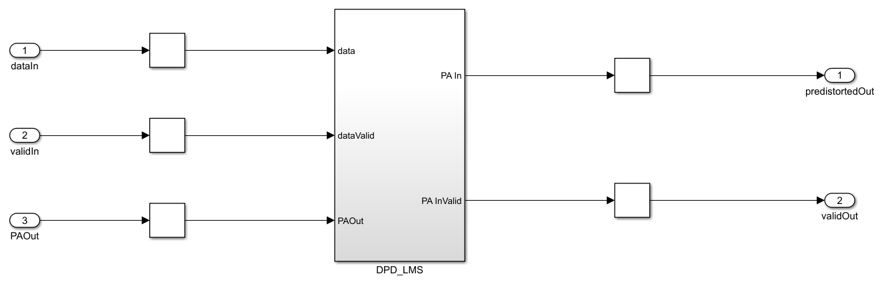### HDL DPD with LMS Coefficients Estimation

The `HDL DPD with LMS` subsystem performs digital predistortion in these stages.

1. Convert 128 Bit I and Q Input Word to 16 Bit Complex Data

The `HDL DPD with LMS` subsystem accepts 128 bit I and Q input words that are generated from the `Baseband OFDM Transmitter` and `RF Subsystem` subsystems. Because the `Digital Predistorter` and the `LMS Coefficient Estimator` subsystems operate on 16 bit complex inputs, the 128 bit I and Q input word data is reconverted to 16 bit complex data using the Repeat block and the `128 to 16 Bit Converter` MATLAB function. The output data sample rate of the `128 to 16 Bit Converter` function is 15.36 MHz (1.92 x 8 MHz).

2. Perform Digital Predistortion with LMS Coefficient Estimation on 16 Bit Complex Data

To perform digital predistortion with LMS coefficients, use the Digital Predistorter and Least Mean Square Coefficient Estimator subsystems. The next couple of sections give a detailed explanation about these subsystems.

3. Convert 16 Bit Complex Data to 128 Bit I and Q Output Word

To generate a 128 bit I and Q output word, a complex 16 bit predistorted signal is grouped into 8 samples and converted into a 128 bit I and Q output word. This operation is performed using the Deserializer1D, Complex to Real-Imag, and Bit Concat Simulink blocks. The output sample rate of data after using the Bit Concat blocks is 1.92 MHz (15.36/8 MHz).

### Digital Predistorter

The `Digital Predistorter` subsystem distorts the 16 bit complex input data using the coefficients that are estimated by the `LMS Coefficient Estimator` subsystem. The DPD design in this example is similar to the HDL Implementation of Digital Predistorter example, which is optimized for memory depth 3 and polynomial degree 3. The input data is placed in a shift register and multiplexed to form a vector based on the memory depth. Then, the vector is concatenated with the nonlinear products of the data depending on the polynomial degree. This concatenation forms a vector of 9 elements, which equals the memory depth times the degree. The dot product of the obtained vector and estimated coefficients provides the predistorted input that is fed as input to the `RF Subsystem` subsystem when you enable the DPDSwitch. Open the `Digital Predistorter` subsystem.

```load_system(modelname); open_system([modelname '/HDL DPD with LMS/DPD_LMS/Digital Predistorter']); ```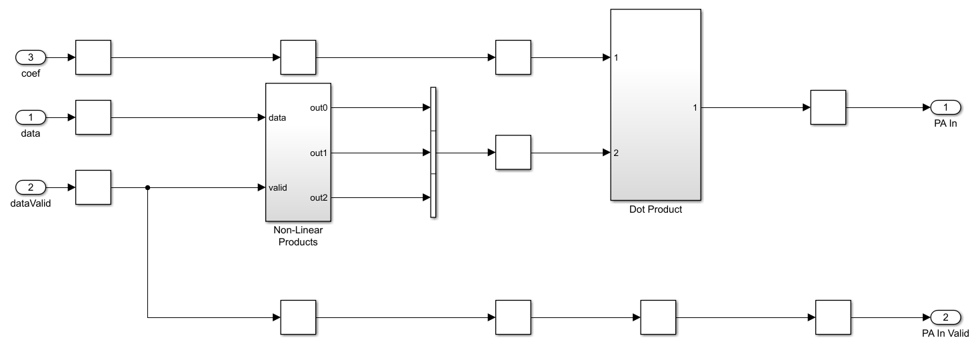### LMS Coefficient Estimator

When PA characteristics vary over time and different operating conditions, using an adaptive estimation algorithm that runs on an FPGA to estimate the inverse of the PA is necessary. In this example, a hardware-friendly estimation algorithm based on the LMS method is considered due to its simple architecture and easier implementation on hardware with less resources compared to other estimation algorithms such as recursive least squares (RLS) and recursive prediction error method (RPEM). The `LMS Coefficient Estimator` subsystem estimates the DPD coefficients from the outputs of the `Digital Predistorter` subsystem (the PA input) and the PA output of the `RF Subsystem` subsystem. Similar to the `Digital Predistorter` subsystem, the `LMS Coefficient Estimator` subsystem also operates at 15.36 MHz. For memory depth 3 and polynomial degree 3, the `LMS Coefficient Estimator` subsystem estimates a total of 9 coefficients. Open the `LMS Coefficient Estimator` subsystem.

```load_system(modelname); open_system([modelname '/HDL DPD with LMS/DPD_LMS/LMS Coefficient Estimator']); ```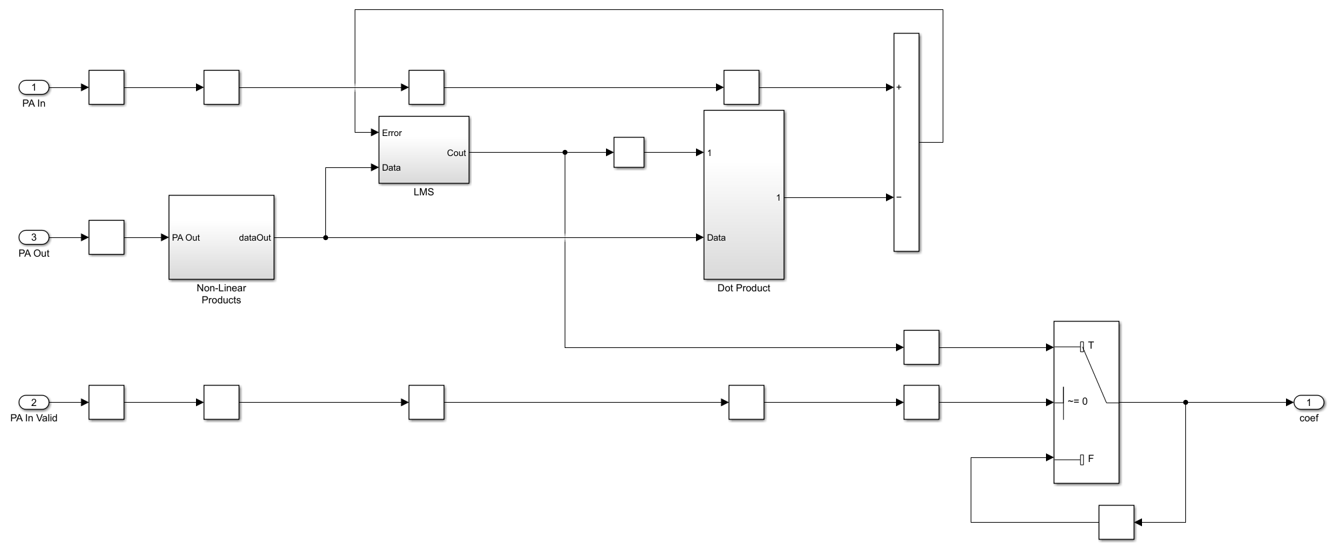The PA output data from the `RF Subsystem` subsystem is placed in a shift register based on the memory depth, which is 3. Then, this vector is concatenated with the nonlinear products of the PA output data depending on the polynomial degree, which is 3. This concatenation forms a vector of 9 elements, which equals the memory depth times the degree. The `LMS` subsystem estimates the coefficients such that the error is minimal between the PA input data and the PA output data. The `Dot product` subsystem performs the dot product of the conjugate of the estimated coefficients and the concatenated PA output data. To send out the estimated coefficients based on PA In Valid, the example uses a switch. When the PA In Valid is high (1), this subsystem sends the current estimated coefficients. Otherwise, the subsystem sends the previously estimated coefficients.

Verification and Results

Run the `HDLDPDwithLMSCoeffExample` model. By default, the DPDSwitch is enabled. If you disable it, the error vector magnitude (EVM) and spectral regrowth in adjacent channels increase. The constellation and spectrum analyzer diagrams show the results of running the `HDLDPDwithLMSCoeffExample` model with the DPD enabled.

```sim(modelname); ```
``` Estimating carrier frequency offset ... First four frames are used for carrier frequency offset estimation. Estimated carrier frequency offset is 1.291716e+00 Hz. Detected and processing frame 5 ------------------------------------------ Header CRC passed Modulation: 16QAM, codeRate=1/2 and FFT Length=128 Data CRC passed Data decoding completed ------------------------------------------ Detected and processing frame 6 ------------------------------------------ Header CRC passed Modulation: 16QAM, codeRate=1/2 and FFT Length=128 Data CRC passed Data decoding completed ------------------------------------------ ```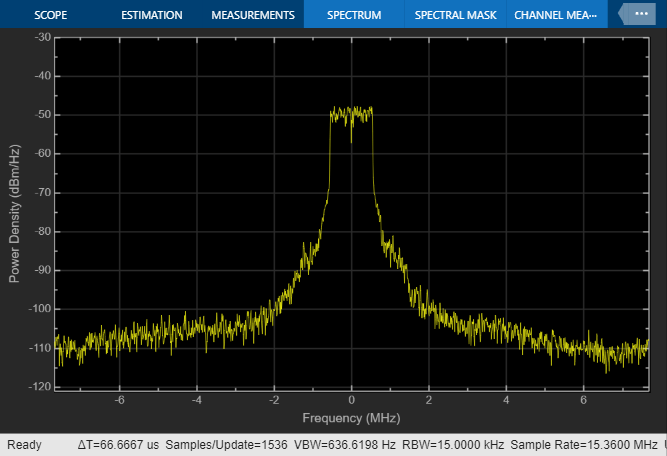HDL Code Generation and Implementation Results

To check and generate HDL code for this model, you must have the HDL Coder™ product. To generate HDL code and a testbench for the `HDL DPD with LMS` subsystem, use the `makehdl` and `makehdltb` commands.

The `HDL DPD with LMS` subsystem is synthesized on the Xilinx® Zynq® Ultrascale RFSoC ZCU111 evaluation board. The frequency obtained after place and route is about 501 MHz. This table displays the post place and route resource utilization results for a 128 bit complex input.

```F = table(... categorical({'Slice LUT'; 'Slice Registers';'DSP'}), ... categorical({'4023'; '10281'; '120'}), ... categorical({'425280'; '850560'; '4272'}), ... categorical({'0.95'; '1.21'; '2.81'}), ... 'VariableNames', ... {'Resources','Utilized','Available','Utilization (%)'}); disp(F); ```
``` Resources Utilized Available Utilization (%) _______________ ________ _________ _______________ Slice LUT 4023 425280 0.95 Slice Registers 10281 850560 1.21 DSP 120 4272 2.81 ```

### DPD with Pre-calculated Coefficients

To perform digital predistortion with pre-calculated coefficients, use the `HDLDPDwithPreCalculatedCoeffExample` model and the `preCalculatedCoeff` MAT file containing pre-calculated coefficients. These coefficients are generated using the `HDLDPDwithLMSCoeffExample` model.

To create a new set of coefficients for any other PA settings, modify the parameters of the PA in the `HDLDPDwithLMSCoeffExample` model according to your requirement and run the model. If you already have a set of estimated coefficients to perform digital predistortion, replace the coefficients in `preCalculatedCoeff` MAT file and run the `HDLDPDwithPreCalculatedCoeffExample` model.

```modelname = 'HDLDPDwithPreCalculatedCoeffExample'; open_system(modelname); ```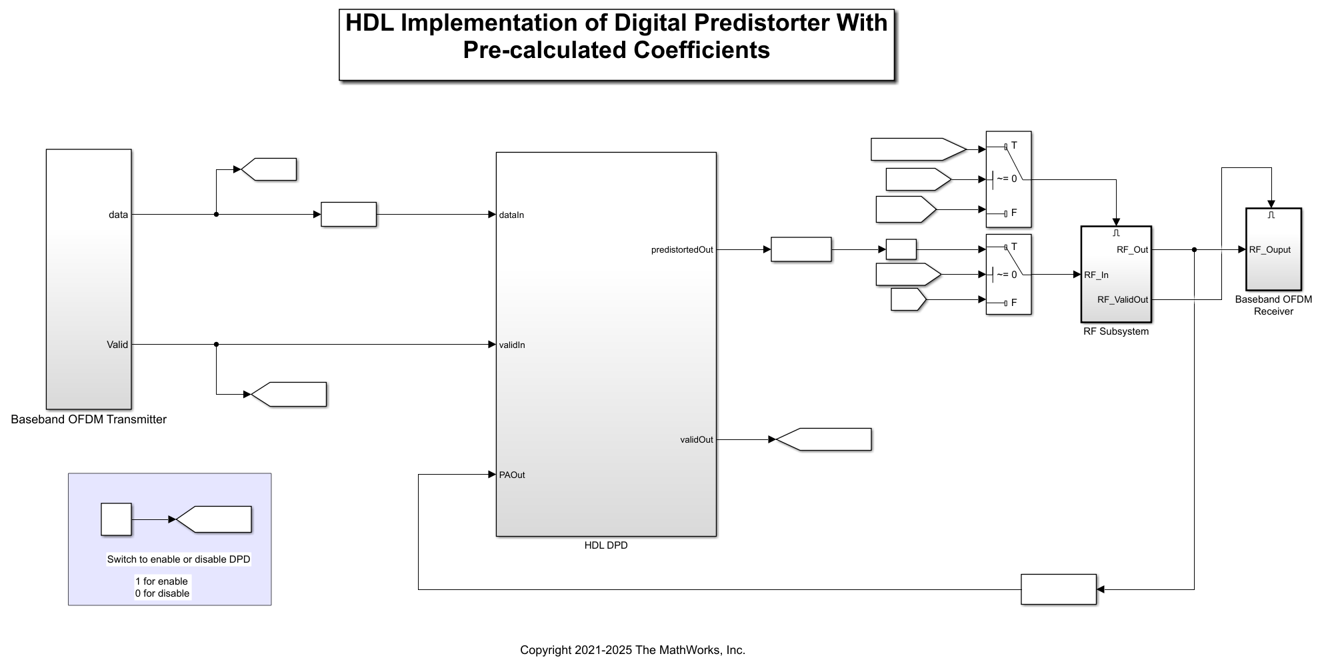Verification and Results

Run the `HDLDPDwithPreCalculatedCoeffExample` model. By default, the DPDSwitch is enabled. If you disable it, the EVM and spectral regrowth in adjacent channels increase. The constellation and spectrum analyzer diagrams show the results of running the `HDLDPDwithPreCalculatedCoeffExample` model with the DPD enabled. You can see that when using DPD with pre-calculated LMS coefficients, the spectrum and constellation remain intact.

```sim(modelname); ```
``` Estimating carrier frequency offset ... First four frames are used for carrier frequency offset estimation. Estimated carrier frequency offset is 1.269763e+00 Hz. Detected and processing frame 5 ------------------------------------------ Header CRC passed Modulation: 16QAM, codeRate=1/2 and FFT Length=128 Data CRC passed Data decoding completed ------------------------------------------ Detected and processing frame 6 ------------------------------------------ Header CRC passed Modulation: 16QAM, codeRate=1/2 and FFT Length=128 Data CRC passed Data decoding completed ------------------------------------------ ```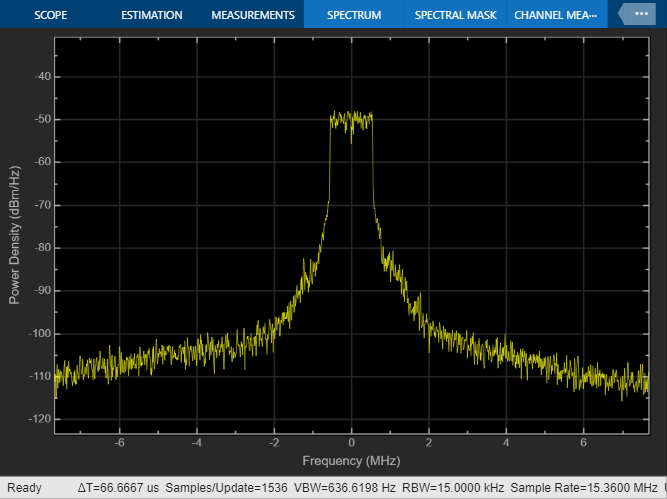HDL Code Generation and Implementation Results

To check and generate HDL code for this model, you must have the HDL Coder™ product. To generate HDL code and a testbench for the `HDL DPD` subsystem, use the `makehdl` and `makehdltb` commands.

The Digital Predistorter with pre-calculated LMS coefficients subsystem is synthesized on a Xilinx® Zynq® Ultrascale RFSoC ZCU011 evaluation board. The frequency obtained after place and route is about 470 MHz. This table displays the post place and route resource utilization results for a 128 bit complex input.

```F = table(... categorical({'Slice LUT'; 'Slice Registers';'DSP'}), ... categorical({'1714'; '4606'; '36'}), ... categorical({'425280'; '850560'; '4272'}), ... categorical({'0.4'; '0.54'; '0.84'}), ... 'VariableNames', ... {'Resources','Utilized','Available','Utilization (%)'}); disp(F); ```
``` Resources Utilized Available Utilization (%) _______________ ________ _________ _______________ Slice LUT 1714 425280 0.4 Slice Registers 4606 850560 0.54 DSP 36 4272 0.84 ```